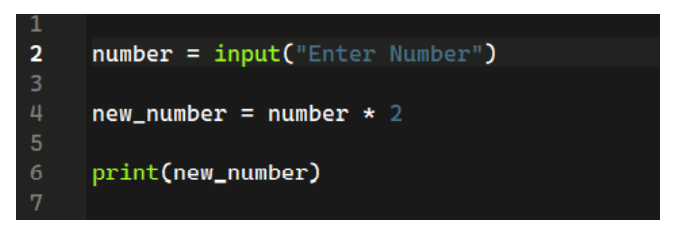# NPTEL The Joy Of Computing Using Python Assignment 2 Answers 2022Are you looking for the Answers to NPTEL The Joy Of Computing Using Python Assignment 2? This article will help you with the answer to the National Programme on Technology Enhanced Learning (NPTEL) Course The Joy Of Computing Using Python . So read the complete article carefully.

## What is The Joy Of Computing Using Python?

The Joy Of Computing Using Python is a fun-filled whirlwind tour of 30 hrs, covering everything you need to know to fall in love with the most sought-after skill of the 21st century. This course includes examples of analytics in a wide variety of industries, and we hope that students will learn how you can use analytics in their careers and life. One of the most important aspects of this course is that you, the student, are getting hands-on experience creating analytics models; we, the course team, urge you to participate in the discussion forums and to use all the tools available to you while you are in the course!

## CRITERIA TO GET A CERTIFICATE

Average assignment score = 25% of the average of best 8 assignments out of the total 12 assignments given in the course.
Exam score = 75% of the proctored certification exam score out of 100

Final score = Average assignment score + Exam score

YOU WILL BE ELIGIBLE FOR A CERTIFICATE ONLY IF THE AVERAGE ASSIGNMENT SCORE >=10/25 AND EXAM SCORE >= 30/75. If one of the 2 criteria is not met, you will not get the certificate even if the Final score >= 40/100.

Below you can find the answers for NPTEL The Joy Of Computing Using Python Assignment 2 Answers

## NPTEL The Joy Of Computing Using Python Assignment 2 Answers 2022:-

Q1. Which statement will print ‘The joy of computing’?

(A) print(The joy of computing)
(B) print The joy of computing printf
(C) (‘The joy of computing’)
(D) print(‘The joy of computing’)

Q2. What is the output of the following code?

(A) Earth is round.
Moon has lot of dust.
(B) Earth is
round.
Moon has
lot of dust.
(C) Earth is
round.
Moon has lot of dust
(D) Earth is round.Moon has lot of dust.

Q3. What should be the syntax of getting age as an input from a user in python?

(A) Age = int(“Enter Age”)
(B) Age = input(“Enter Age”)
(C) Age = get(“Enter Age”)
(D) Age = input(Enter Age)

???? Next Week Answers: Assignment 03 ????

Q4. What should be the value of _ to print all numbers from 0-10?

(A) 10
(B) 9
(C) 11
(D) None of the above

Q5. What will be the output of the following code?

(A) 0
(B) 45
(C) 43
(D) 50

Q6. What will be the output? suppose the input is 20(A) 40
(B) Error
(C) 2020
(D) None of the above

Q7. What will be the output of the following code be?

(A) number is less than 0
(B) number is greater than 0
(C) number is zero
(D) Error

Q8. What will be the output of the following code?

(A) 9
(B) 10
(C) 0
(D) None of the above

Q9. What will be the output of the following code?

(A) 56
(B) 45
(C) 0
(D) Error

Q10. What will be the output of the following code?

(A) 1,2,3,4,5,6,7,8,9,10
(B) 2,4,6,8,10
(C) 0,1,2,3,4,5,6,7,8,9,10
(D) Error

## Programming Assigment week 2

Q1. Take an integer input from user and print the same number.

Code:-

``````a = int(input())
print(a)``````

Q2. Take two integers as an input and display the difference of second number from first number.

Code:-

``````a = int(input())
b = int(input())
print(a-b)``````

Q3.You given a number as the an input. You have to display all the numbers from 0 till x.

Code:-

``````x = int(input())
for x in range(0,x+1):
print(x)``````